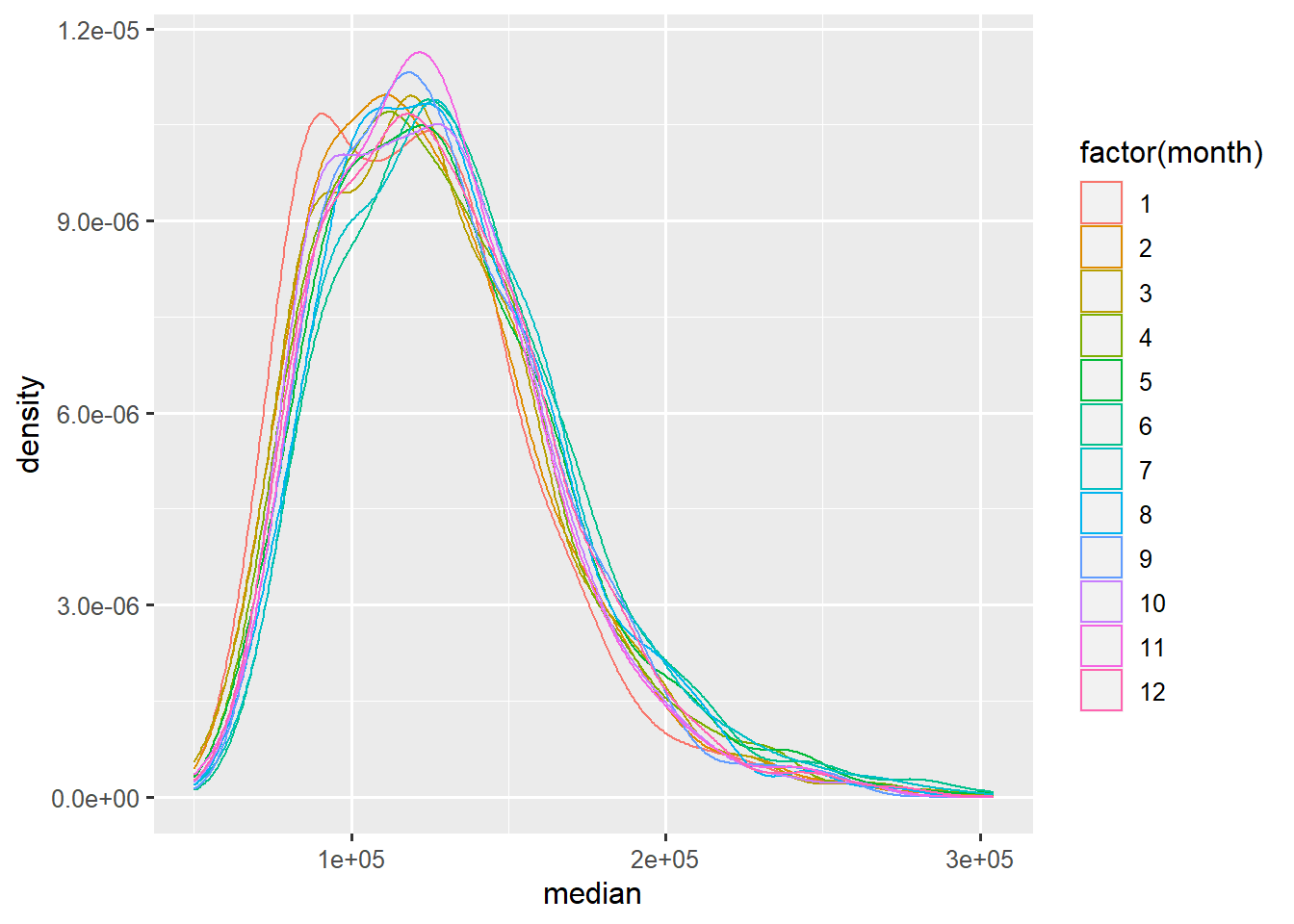# Background

## Purpose of this seminar

This seminar introduces how to use the R `ggplot2` package, particularly for producing statistical graphics for data analysis.

• First the underlying grammar (system) of graphics is introduced with examples.
• Then, we’ll practice using the elements of the grammar by creating a customized graph.
• Finally, we’ll address common issues that arise when creating statistical graphics.

`Text in this font` signifies `R` code or variables in a data set

Text that appears like this represents an instruction to practice `ggplot2` coding

## Seminar packages

Next we load the packages into the current `R` session with `library()`. In addition to `ggplot2`, we load package `MASS` (installed with `R`) for data sets.

``````#load libraries into R session
library(ggplot2)
library(MASS)``````

Please use `library()` to load packages `ggplot2` and `MASS`.

## The `ggplot2` package

• produces layered statistical graphics
• uses an underlying “grammar” to build graphs layer-by-layer rather than providing premade graphs
• is easy enough to use without any exposure to the underlying grammar, but is even easier to use once you know the grammar
• allows the user to build a graph from concepts rather than recall of commands and options

## ggplot2 documentation

https://ggplot2.tidyverse.org/reference/

The official reference webpage for `ggplot2` has help files for its many functions an operators. Many examples are provided in each help file.

# The grammar of graphics

## What is a grammar of graphics?

A grammar of a language defines the rules of structuring words and phrases into meaningful expressions.

A grammar of graphics defines the rules of structuring mathematic and aesthetic elements into a meaningful graph.

Leland Wilkinson (2005) designed the grammar upon which `ggplot2` is based.

## Elements of grammar of graphics

1. Data: variables mapped to aesthetic features of the graph.
2. Geoms: objects/shapes on the graph.
3. Stats: statistical transformations that summarize data,(e.g mean, confidence intervals).
4. Scales: mappings of aesthetic values to data values. Legends and axes visualize scales.
5. Coordinate systems: the plane on which data are mapped on the graphic.
6. Faceting: splitting the data into subsets to create multiple variations of the same graph (paneling).

## The `Sitka` dataset

To practice using the grammar of graphics, we will use the `Sitka` dataset (from the `MASS` package).

Note: Data sets that are loaded into `R` with a package are immediately available for use. To see the object appear in RStudio’s `Environment` pane (so you can click to view it), run `data()` on the data set, and then another function like `str()` on the data set.

Use `data()` and then `str()` on `Sitka` to make it appear in the Environment pane.

The `Sitka` dataset describes the growth of trees over time, some of which were grown in ozone-enriched chambers. The data frame contains 395 rows of the following 4 columns:

• size: numeric, log of size (height times diameter2)
• Time: numeric, time of measurement (days since January 1, 1988)
• tree: integer, tree id
• treat: factor, treatment group, 2 levels=“control” and “ozone”

Here are the first few rows of `Sitka`:

size Time tree treat
4.51 152 1 ozone
4.98 174 1 ozone
5.41 201 1 ozone
5.90 227 1 ozone
6.15 258 1 ozone
4.24 152 2 ozone

## The `ggplot()` function and aesthetics

All graphics begin with specifying the `ggplot()` function (Note: not `ggplot2`, the name of the package)

In the `ggplot()` function we specify the data set that holds the variables we will be mapping to aesthetics, the visual properties of the graph. The data set must be a `data.frame` object.

Example syntax for `ggplot()` specification (`italicized` words are to be filled in by you):

`ggplot(data, aes(x=xvar, y=yvar))`

• `data`: name of the `data.frame` that holds the variables to be plotted
• `x` and `y`: aesthetics that position objects on the graph
• `xvar` and `yvar`: names of variables in `data` mapped to `x` and `y`

Notice that the aesthetics are specified inside `aes()`, which is itself nested inside of `ggplot()`.

The aesthetics specified inside of `ggplot()` are inherited by subsequent layers:

``````# scatter plot of volume vs sales
ggplot(txhousing, aes(x=volume, y=sales)) +
geom_point() ``````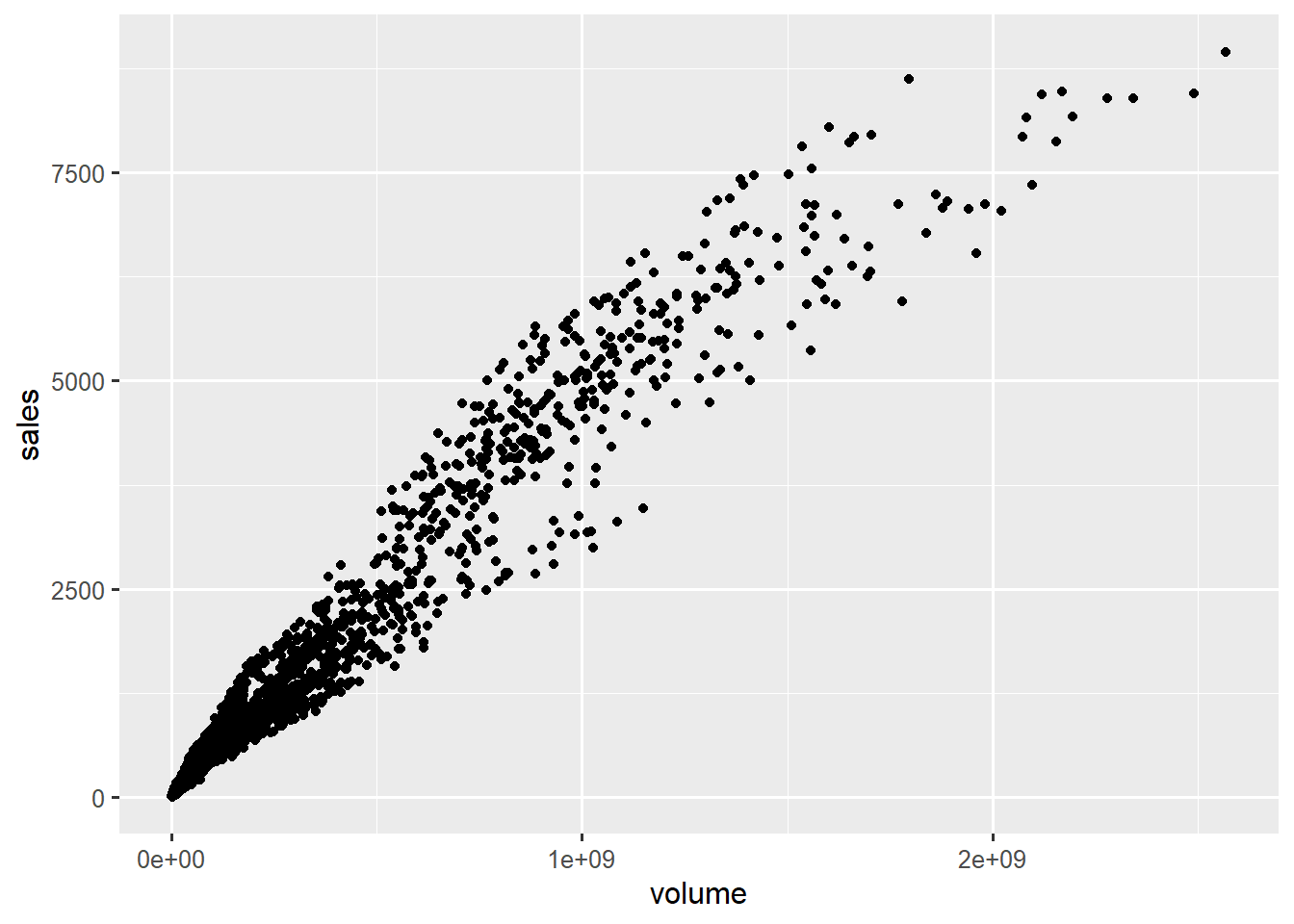geom_point() inherits x and y aesthetics

Initiate a graph of `Time` vs `size` by mapping `Time` to `x` and `size` to `y` from the data set `Sitka`.

Without any additional layers, no data will be plotted.

## Layers and overriding aesthetics

Specifying just `x` and `y` aesethetics alone will produce a plot with just the 2 axes.

``ggplot(data = txhousing, aes(x=volume, y=sales))``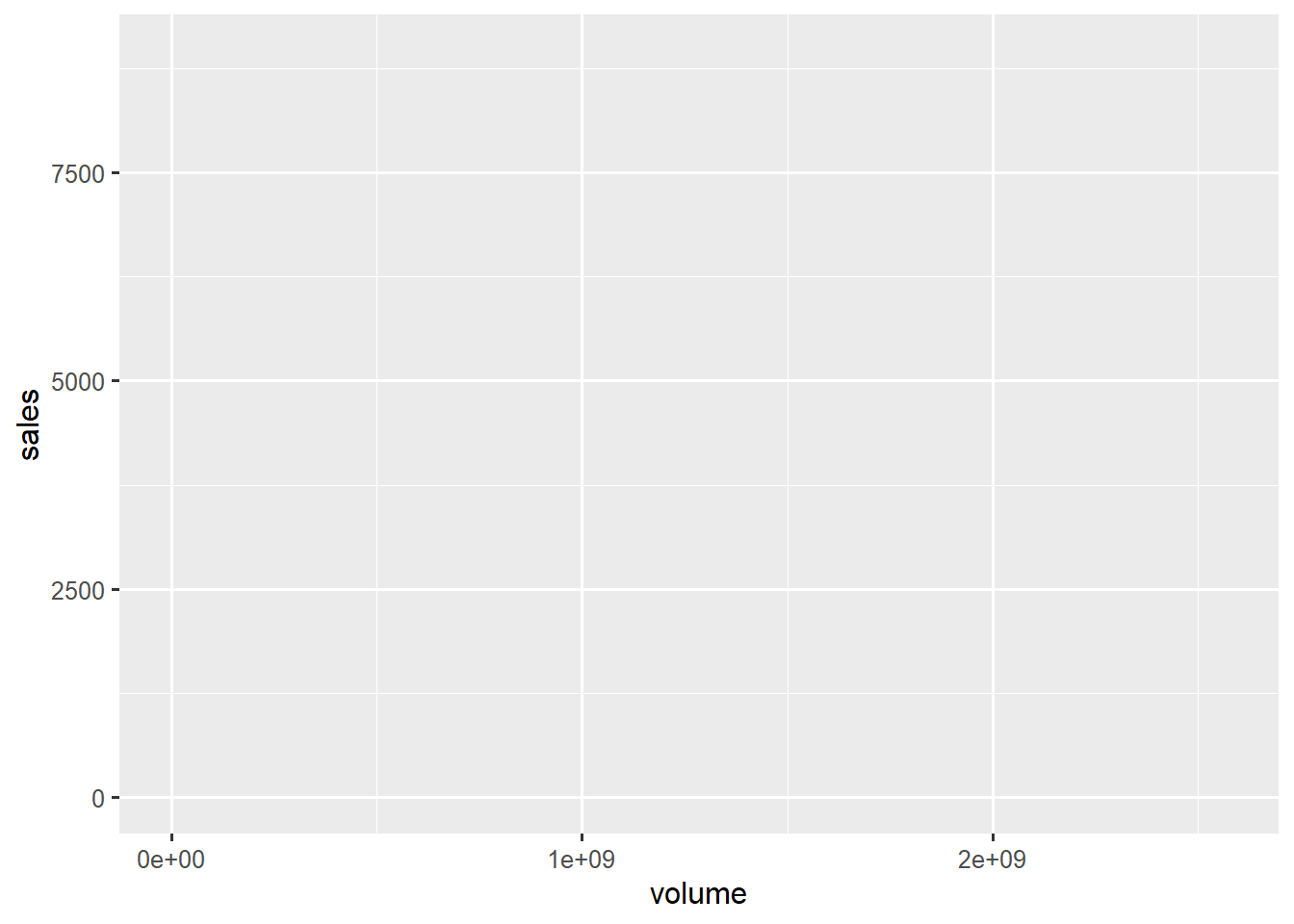without a geom or stat, just axes

We add layers with the character `+` to the graph to add graphical components.

Layers consist of geoms, stats, scales, and themes, which we will discuss in detail.

Remember that each subsequent layer inherits its aesthetics from `ggplot()`. However, specifying new aesthetics in a layer will override the aesthetics speficied in `ggplot()`.

``````# scatter plot of volume vs sales
#  with rug plot colored by median sale price
ggplot(txhousing, aes(x=volume, y=sales)) +     # x=volume and y=sales inherited by all layers
geom_point() +
geom_rug(aes(color=median))   # color will only apply to the rug plot because not specified in ggplot()``````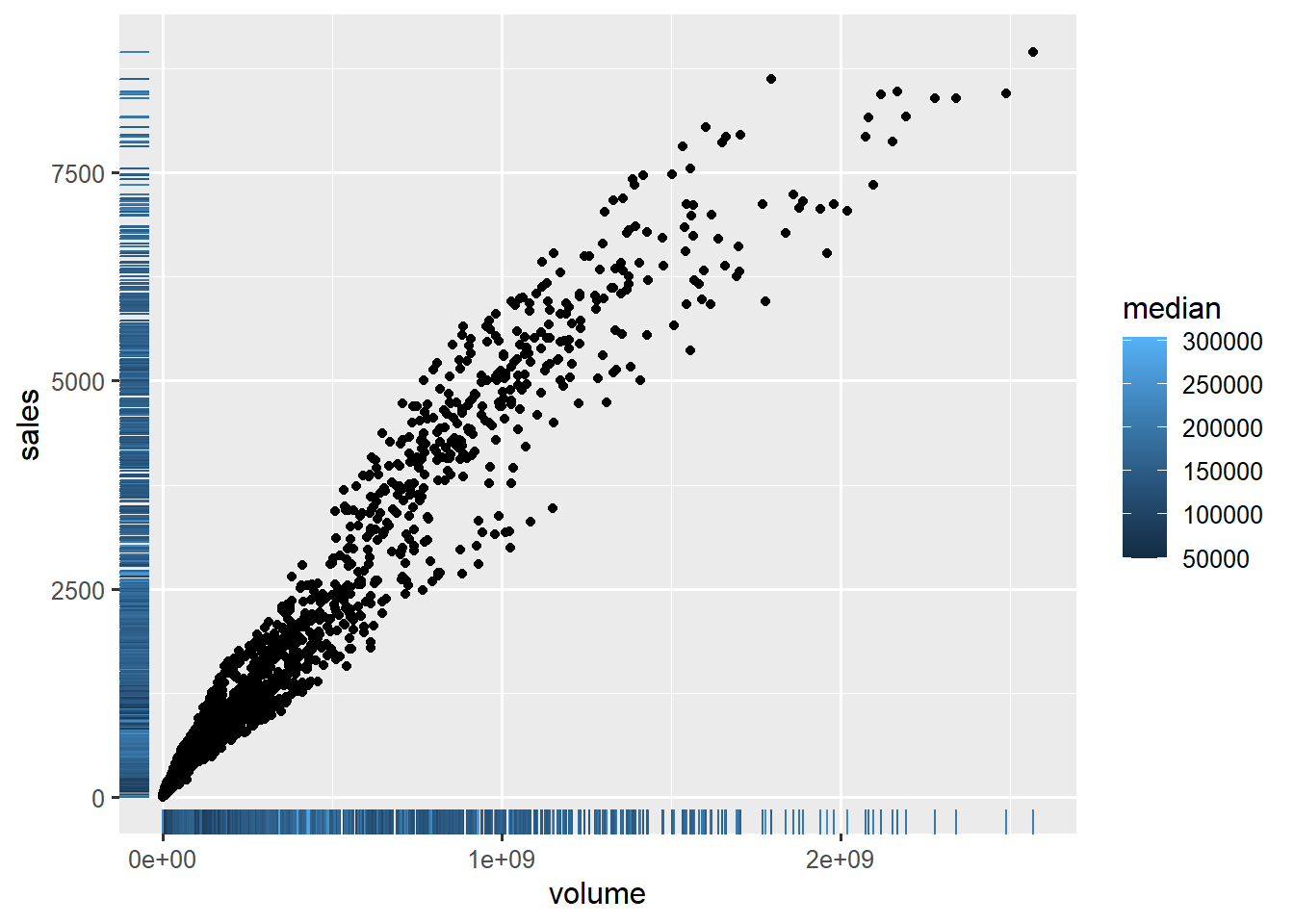both geoms inherit aesthetics from gglot, but geom_rug() also adds color aesthetic

Add a `geom_point()` layer to the `Sitka` graph we just initiated.

Add an additional `geom_smooth()` layer to the graph.

Both geom layers inherit `x` and `y` aesthetics from `ggplot()`.

Specify `aes(color=treat)` inside of `geom_point()`.

Notice that the coloring only applies to `geom_point()`.

## Aesthetics

Aesthetics are the visual properties of objects on the graph.

Which aesthetics are required and which are allowed vary by geom.

Commonly used aesthetics:

• `x`: positioning along x-axis
• `y`: positioning along y-axis
• `color`: color of objects; for 2-d objects, the color of the object’s outline (compare to fill below)
• `fill`: fill color of objects
• `linetype`: how lines should be drawn (solid, dashed, dotted, etc.)
• `shape`: shape of markers in scatter plots
• `size`: how large objects appear
• `alpha`: transparency of objects (value between 0, transparent, and 1, opaque – inverse of how many stacked objects it will take to be opaque)

Change the aesthetic `color` mapped to `treat` in our previous graph to `shape`.

## Mapping vs setting

Map aesthetics to variables inside the `aes()` function. By mapping, we mean the aesthetic will vary as the variable varies. For example, mapping `x=time` causes the position of the plotted data to vary with values of variable “time”. Similary, mapping `color=group` causes the color of objects to vary with values of variable “group”.

``````# mapping color to median inside of aes()
ggplot(txhousing, aes(x=volume, y=sales)) +
geom_point(aes(color=median))``````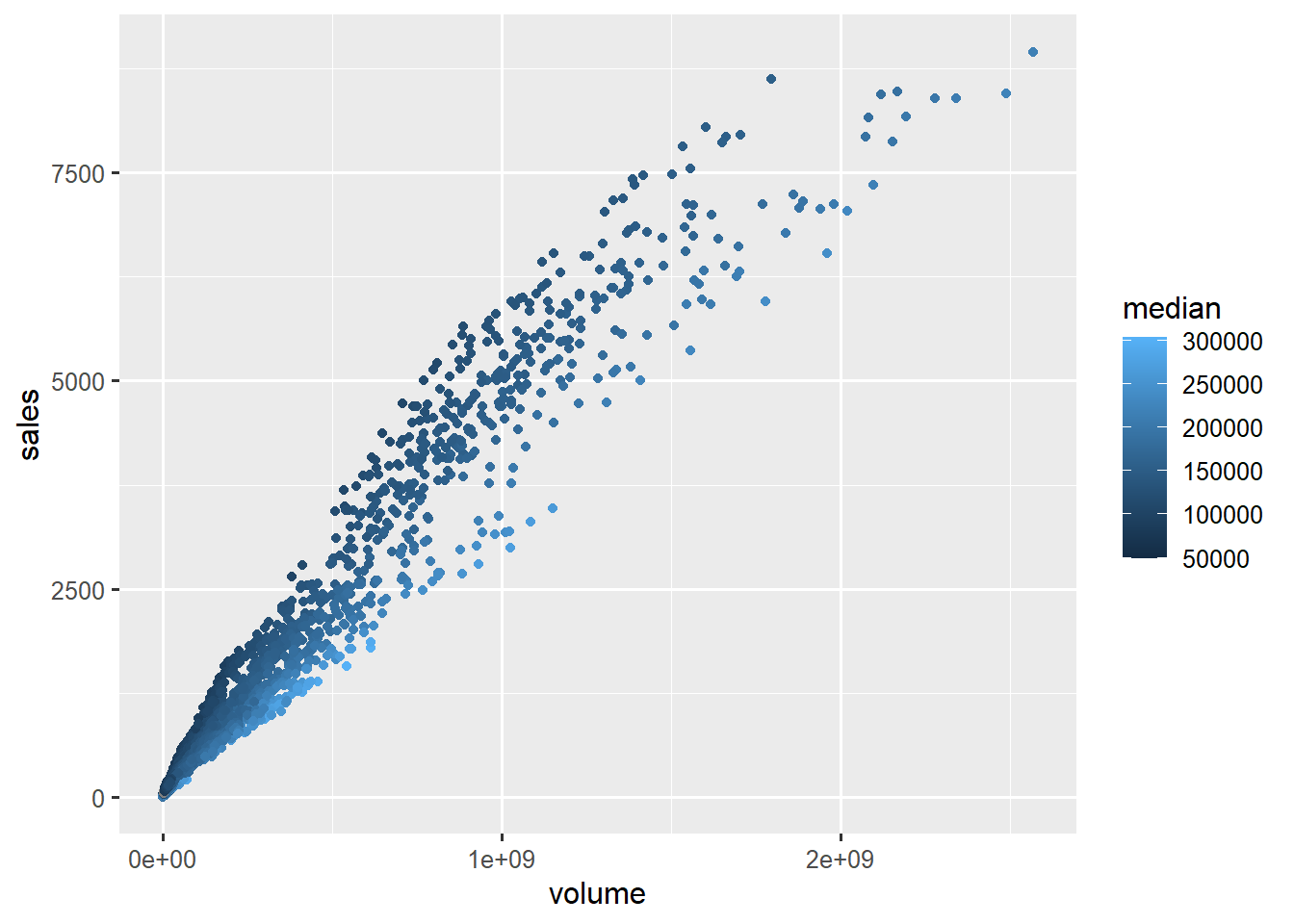color of points varies with median price

Set aesthetics to a constant outside the `aes()` function.

Compare the following graphs:

``````# setting color to green outside of aes()
ggplot(txhousing, aes(x=volume, y=sales)) +
geom_point(color="green")``````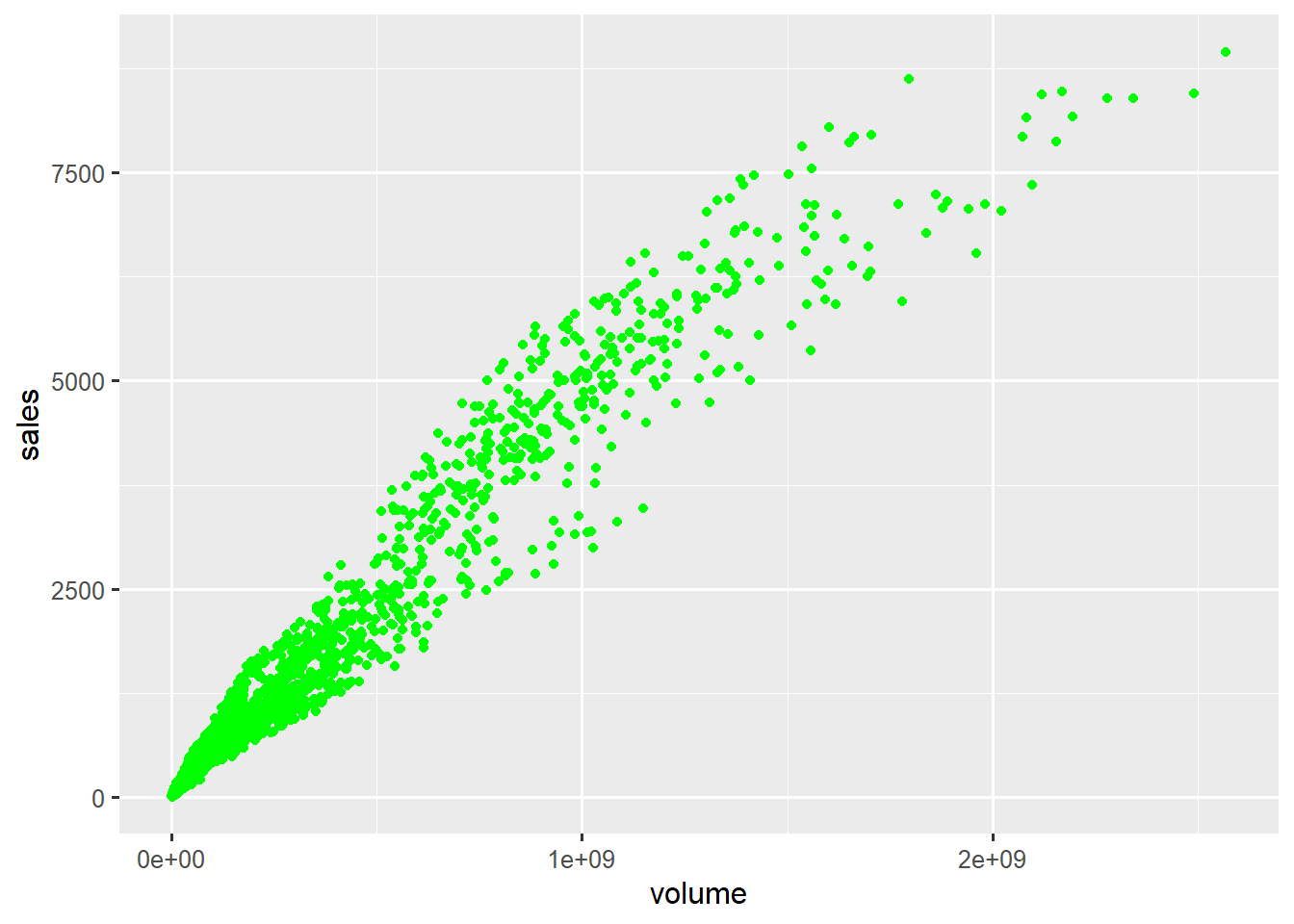color of points set to constant green

Create a new graph for data set `Sitka`, a scatter plot of `Time` (x-axis) vs `size` (y-axis), where all the points are colored “green”.

Setting an aesthetic to a constant within `aes()` can lead to unexpected results, as the aesthetic is then set to a default value rather than the specified value.

``````# color="green" inside of aes()
# geom_point() cannot find a variable called "green" and
#   uses a default color instead
ggplot(txhousing, aes(x=volume, y=sales)) +
geom_point(aes(color="green"))``````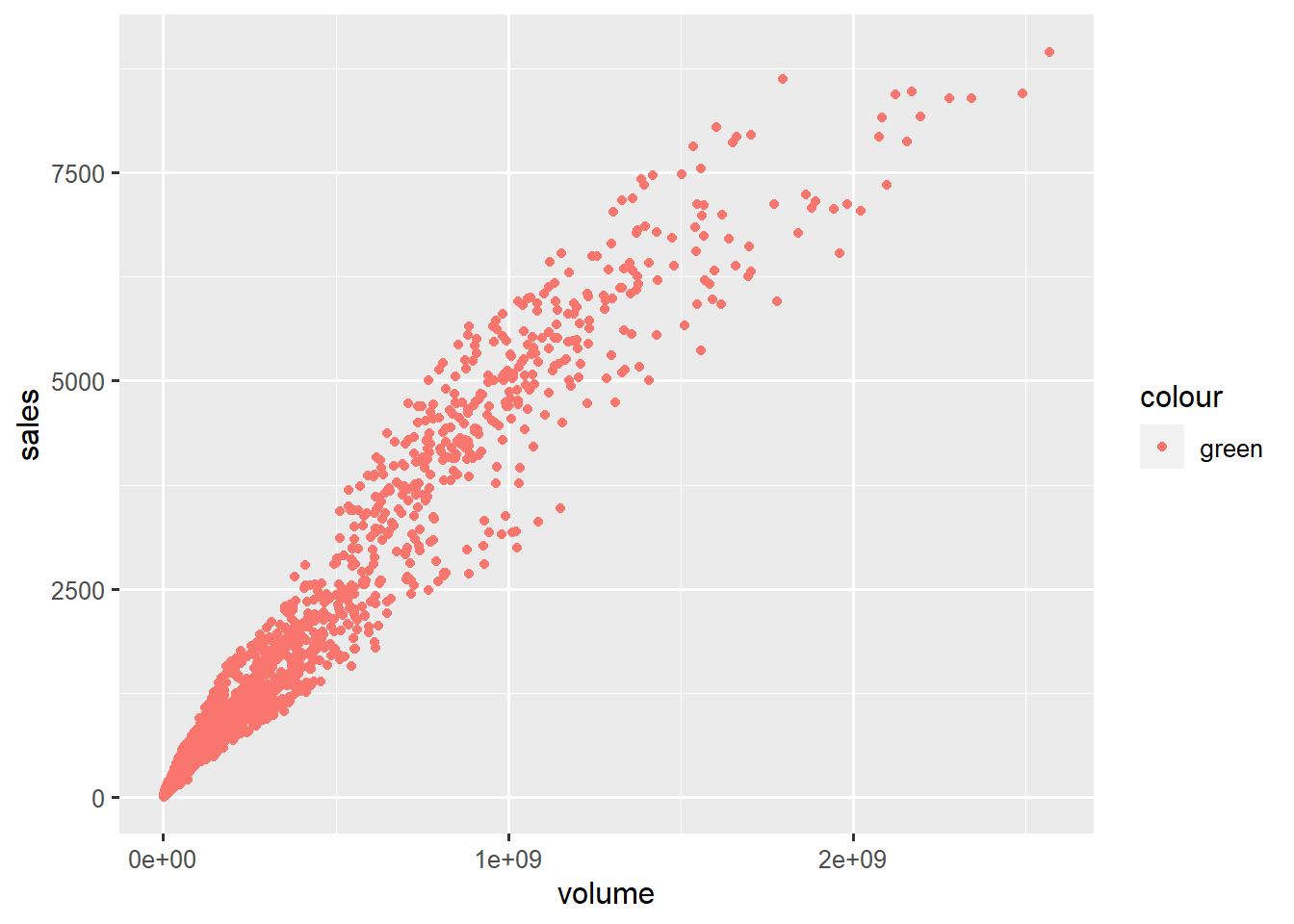aesthetic set to constant within aes() leads to unexpected results

## Geoms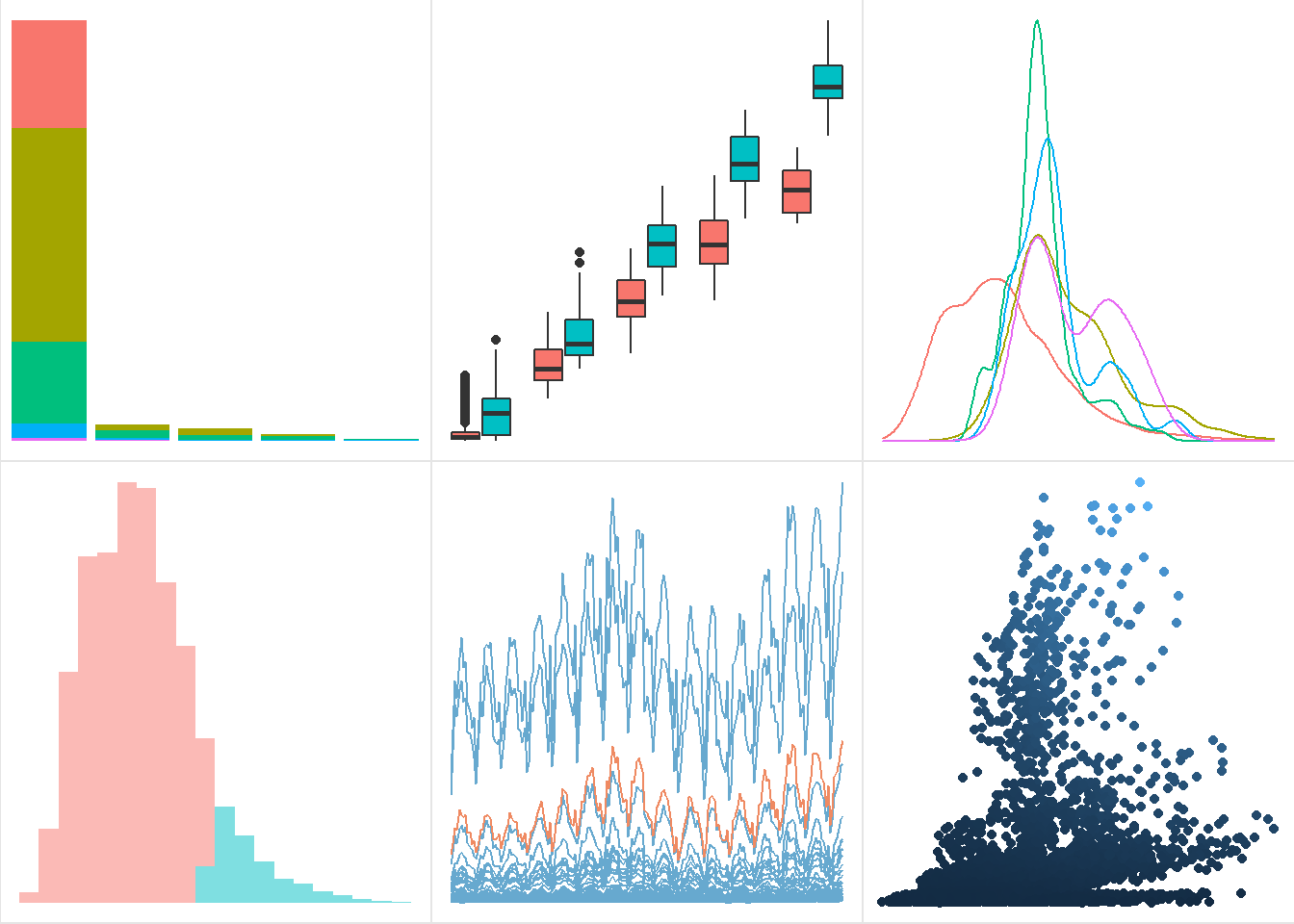geoms: bar, boxplot, density, histogram, line, point

Geom functions differ in the geometric shapes produced for the plot.

Some example geoms:

• `geom_bar()`: bars with bases on the x-axis
• `geom_boxplot()`: boxes-and-whiskers
• `geom_errorbar()`: T-shaped error bars
• `geom_density()`: density plots
• `geom_histogram()`: histogram
• `geom_line()`: lines
• `geom_point()`: points (scatterplot)
• `geom_ribbon()`: bands spanning y-values across a range of x-values
• `geom_smooth()`: smoothed conditional means (e.g. loess smooth)
• `geom_text()`: text

## Geoms and aesthetics

Each geom is defined by aesthetics required for it to be rendered. For example, `geom_point()` requires both `x` and `y`, the minimal specification for a scatterplot.

Geoms differ in which aesthetics they accept as arguments. For example, `geom_point()` accepts the aesthetic `shape`, which defines the shapes of points on the graph, while `geom_bar()` does not accept `shape`.

Check the geom function help files for required and understood aesthetics. In the Aesthetics section of the geom’s help file, required aesthetics are bolded.

We will tour some commonly used geoms.

## Histograms

``````ggplot(txhousing, aes(x=median)) +
geom_histogram() ``````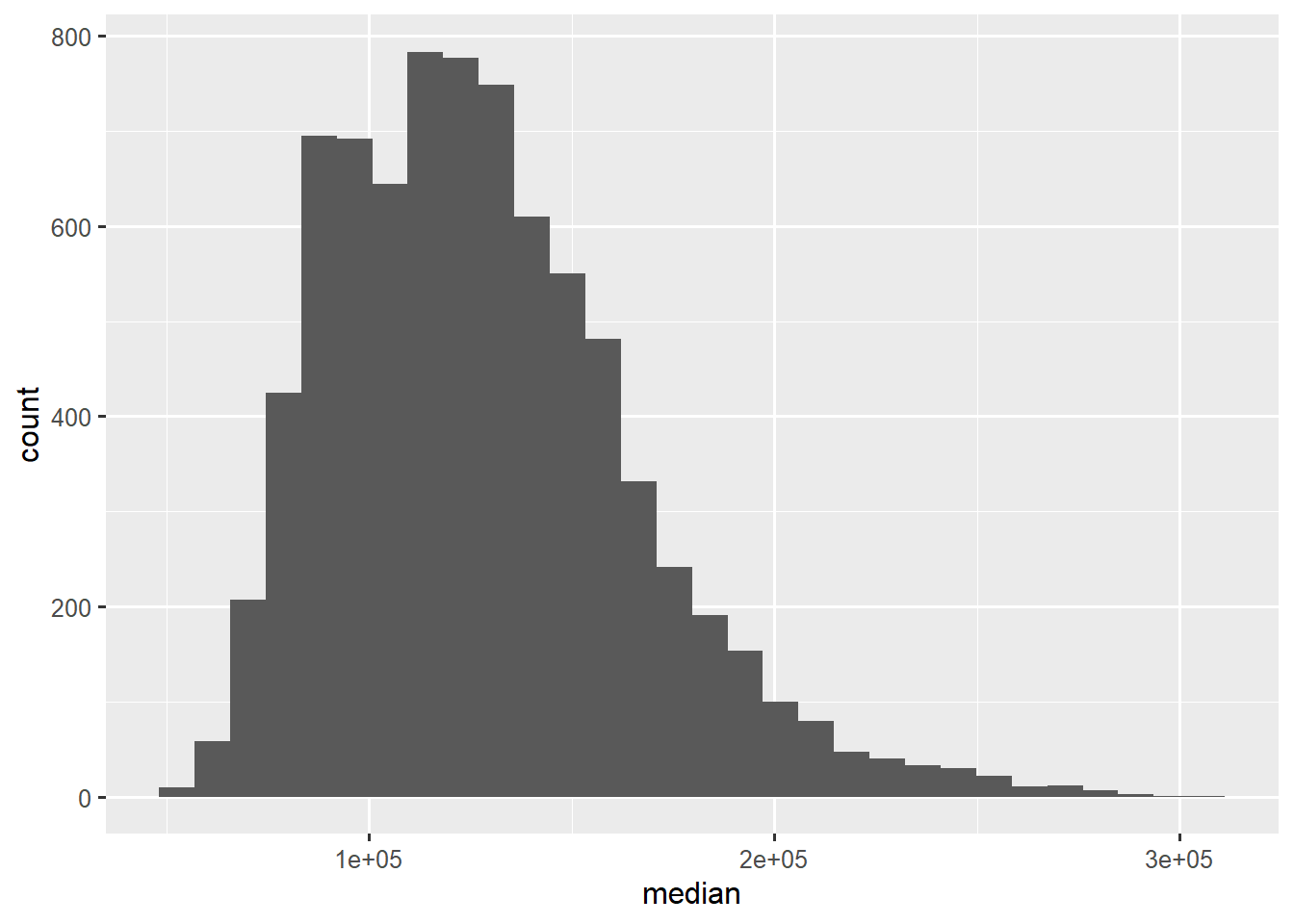histograms visualize distribution of variable mapped to x

Histograms are popular choices to depict the distribution of a continuous variable.

`geom_histogram()` cuts the continuous variable mapped to `x` into bins, and count the number of values within each bin.

Create a histogram of `size` from data set `Sitka`.

`ggplot2` issues a message urging you to pick a number of bins for the histogram (it defaults to 30), using the `bins` argument.

Specify `bins=20` inside of `geom_histogram()`. Note: `bins` is not an aesthetic, so should not be specified within `aes()`.

## Density plots

``````ggplot(txhousing, aes(x=median)) +
geom_density() ``````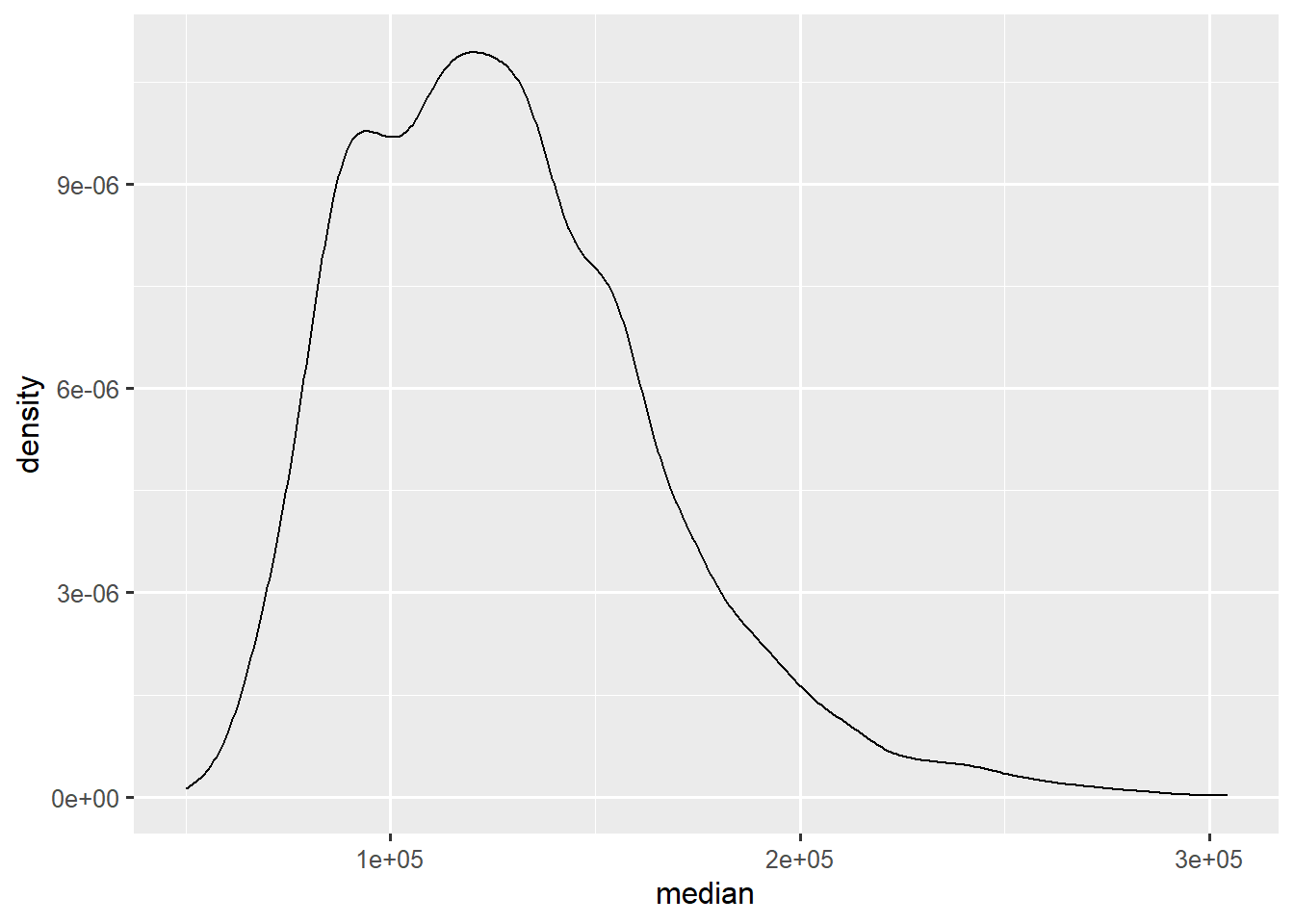density plots visualize smoothed distribution of variable mapped to x

Denisty plots are basically smoothed histograms.

Density plots, unlike histograms, can be plotted separately by group by mapping a grouping variable to `color`.

``````ggplot(txhousing, aes(x=median, color=factor(month))) +
geom_density() ``````